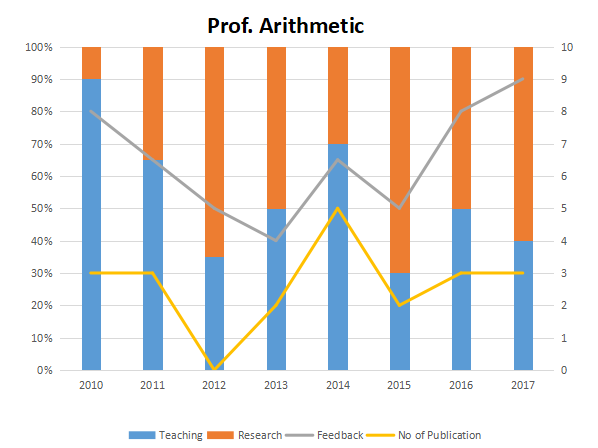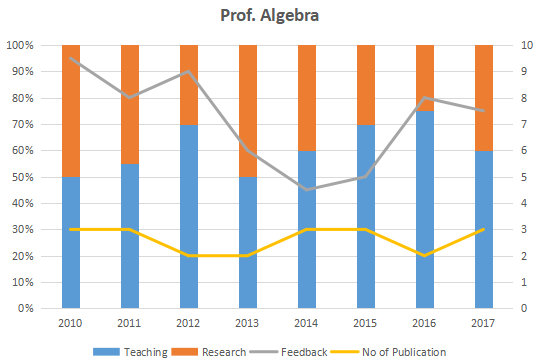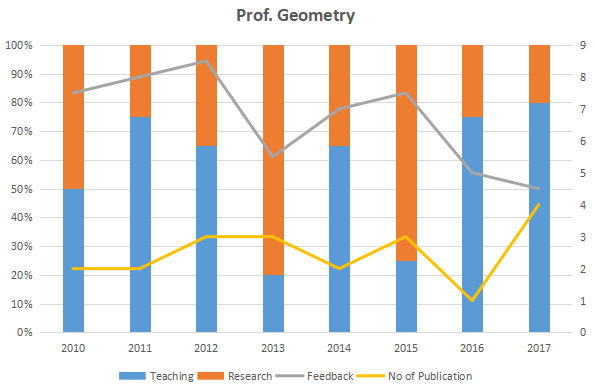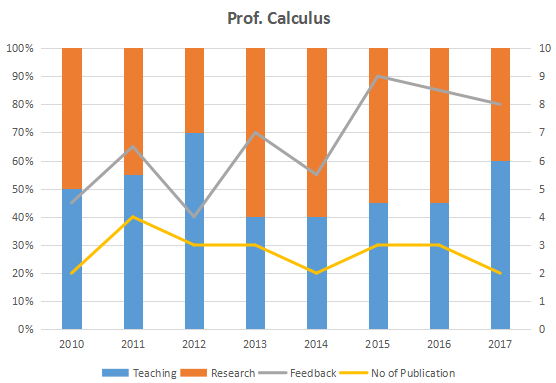### XAT 2018 Question 72

Instructions

The graph below represent the performance of four professors, across years. measured on four variables: Percentage of time spent on research, teaching, feedback on (a scale of 10. right-hand side) and number of publications (right-hand side). Assume that the cumulative time spent per year on research and teaching activities are sane for all four professors and each of them taught only one course of 90 classroom hours.Question 72

# Which of the following, shows the maximum year to year percentage growth in feedback?

Solution

Let the required percentage be P.

Option A: PA = (6.5 - 4) * 100/ 4 = 62.5%

Option B: PB = (8 - 5) * 100/ 5 = 60%

Option C: PC = (7 - 4) * 100/ 4 = 75%

Option D: PD = (9 - 5.5) * 100/ 5.5 = 63.63%

Thus, option C shows the maximum year-on-year growth and is the answer.